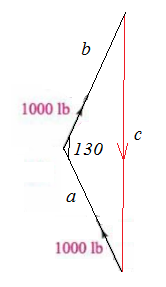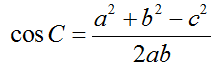Question
1 views

Tugboat towing a barge in figure has a tension of 100lb on each cable. What is the magnitude of the resultant force excerted by the tug? Add ther two force vectors to create a triangle and find the third side.

This uses Law of Cosines & Vectors

check_circle

Step 1

Given:

Tension in both cables (T) = 1000 lb

angle between both cables θ = 50o

Step 2

vector diagram will be,the vectors are rearranged in manner so that the end of one vector meets tail of another vector.

Step 3

Angle between both vector will be = 180-50 = 130.

With the help of following cosine formula magnitude of c can be calculated....

### Want to see the full answer?

See Solution

#### Want to see this answer and more?

Solutions are written by subject experts who are available 24/7. Questions are typically answered within 1 hour.*

See Solution
*Response times may vary by subject and question.
Tagged in

### Physics